# Activation Function

## Neuron Structure

An artificial neuron is composed of an adder and an activation function. The input signals (represented by x in the figure) are multiplied by each weight W. Then, the summation y1 is applied to the activation function f(y1) to produce the output z1.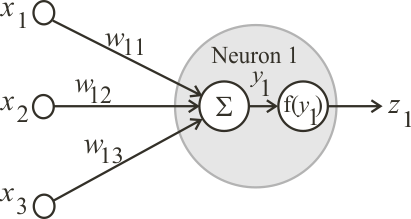Problem 1 Indicate what is the purpose of the weights? For instance, what would happen, if one weight is very big compared to the other weigths?

## Activation Function

The activation function must be a sigmoid (has an S shape), real, continuous, limited and have a positive derivative. Two of the most popular activation functions (for multilayer neural networks) are the hyperbolic tangent (z = tanh(y)) and the logistic function (z = logsig(y)). The ANN shown below has three inputs and one output.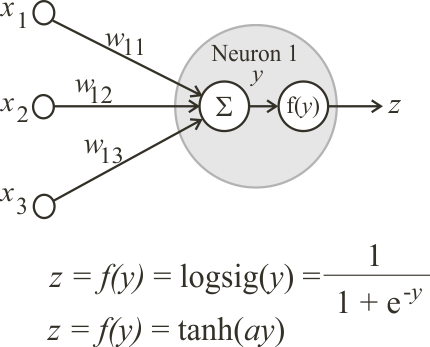## Bias

Each neuron has a fixed input called Bias that always has a value of one. The bias allows the neuron to increase its learning flexibility by shifting the value of y as required for the specific conditions of the problem.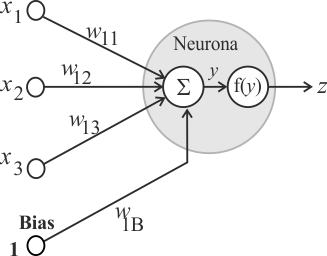Problem 2 What would happen if the ANNs did not have any Bias (fixed input)? For instance, suppose that y must be five, when all the inputs are zero.

 Problem 3 Write the Neural Lab code to plot the logistic function: z = logsig(y).

 Solution 3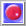Neural Lab open Neural Lab and click on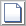New Project. Set the project name to Logsig and select the Main File only option. Then write the code show below. The code begins by creating a vector y of length 100 with values from -10.0 to 10.0. Then, another vector z is computed using the equation of the logsig function. The code ends by creating a XyChart that will be displayed and saved to disk.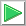Run press the button to run the code. If you do not have any errors, the variables y and z will be displayed in the variable list and the graph will be displayed.

 Logsig\Main.lab Vector y; y.CreateSeries(-10.0, 10.0, 100); Vector z; z = 1.0 / (1.0 + exp(-1.0*y)); //________________________________________ Create XY Chart XyChart chart; chart.AddGraph(y, z, "logsig", 2, 1, 0, 255, 0); chart.SetLimits(-10.0, 10.0, 0, 1.0); chart.SetColorMode(2); chart.SaveAndShow();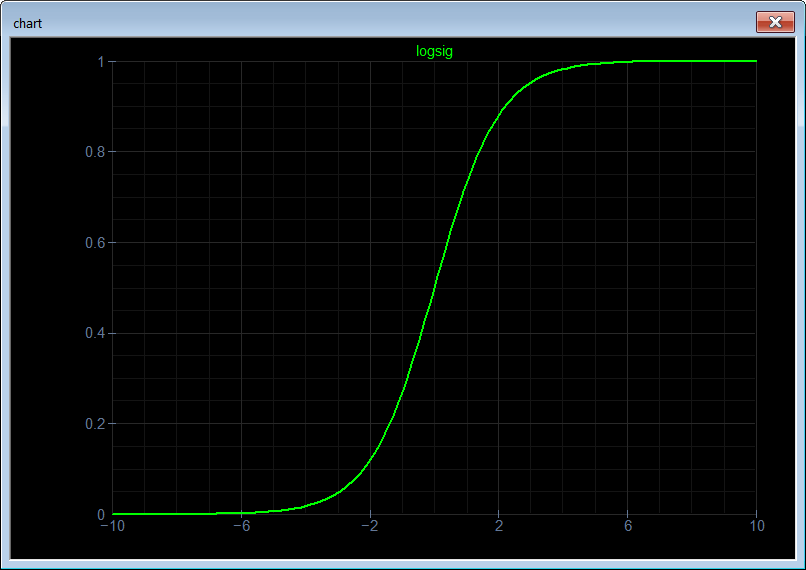Problem 4 Write the Neural Lab code to plot the tanh function: z = tanh(ay) . Use a value of 1.5 for the constant a.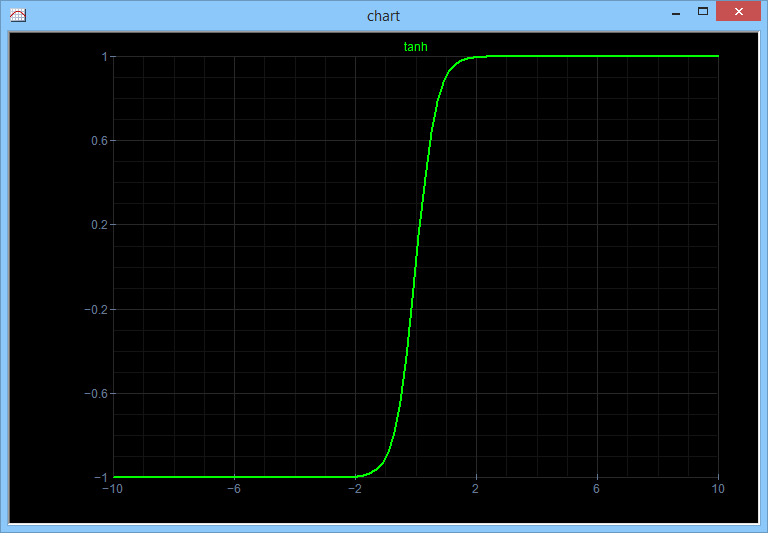Problem 5 Indicate the main similarities and differences between the functions: logsig and tanh.

 Problem 6 Indicate whether the next statement is true or false. When the neurons of an ANN use the logsig function the output values of the network cannot exceed a value of one no matter what input is applied to the network. A value outside the range [0 1] is impossible to attain at the output of the ANN. If the required range is bigger than from zero to one, then the output signal must be scaled appropriately.

 Problem 7 Indicate whether the next statement is true or false. When the neurons of an ANN operate using the tanh function, it is very important to note that the output signal is limited to the range from -1 to 1. The tanh function has a shape very similar to the shape of the logsig function. However, the lower range for the logsig function is zero when compared with the lower range of the tanh which is -1.

 Tip Always scale the output of an ANN using the logsig function to efficiently use the output range (0 to 1). This means that if there are some occasional big values that will force the scaling to attenuate severely the output signal, these values must be clipped before scaling.

 Tip In some applications, it is very important to compute quickly the activation function of an artificial neuron. This can be accomplished by: Building a lookup table with the values of the activation function for a set of values uniformly distributedBuilding a lookup table with the values of the activation function derivative for a set of values uniformly distributedMaking use of the properties of the activation function. For instance, if the input value is bigger than 5, the activation function is one. If the input value is less than -5, the activation function is 0 for the logsig(y) and -1 for the tanh(y) .

 Problem 8 Show that the derivative of the logsig function z=logsig(y) can be expressed as z' = z [1 - z].

 Problem 9 Show that the derivative of the tanh function z=tanh(ay) can be expressed as z' = a [1 - z2].

 Problem 10 Compute manually the output value of the neural network shown below. Suppose that the neuron is using the logsig function.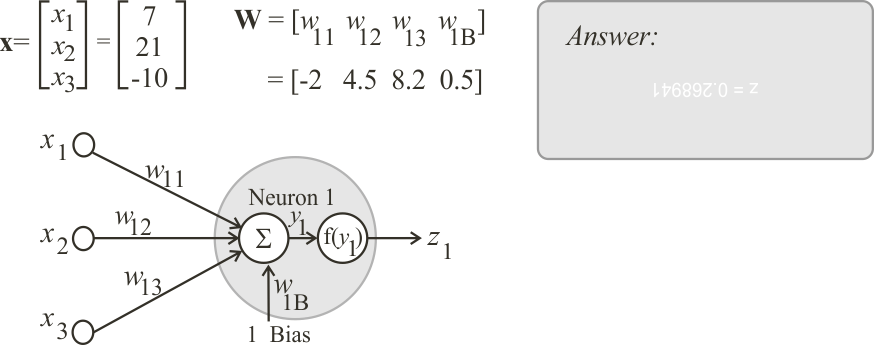Problem 11 Repeat the last problem using Neural Lab code.

 Solution 11New Project click the button to create a new project. Set the project name to ComputOutput and select the Main File only option. Write the code shown below.Run click the button to execute the code. If you do not have any errors, the variables x, W, y and z will be displayed in the variable list.

 ComputeOutput\Main.lab //_________________________________________ Main File Vector x; x.Create(4); x = 7.0; x = 21.0; x = -10.0; x = 1.0; Matrix W; W.Create(1, 4); W = -2.0; W = 4.5; W = 8.2; W = 0.5; Vector y = W*x; double z = 1.0 / (1.0 + exp(-1.0*y));

 Problem 12 Find an expression for the output value z1 of the neural network shown below. The ANN has n inputs, one neuron in the output layer and one output. Suppose that the neuron uses the logsig function.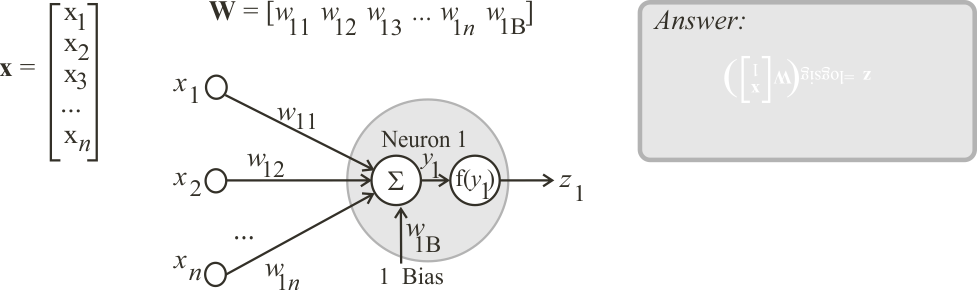Problem 13 Find an expression for the output value of the neural network shown below. The ANN has two inputs, two neurons in the output layer and two outputs. Suppose that the ANN is using the logsig function.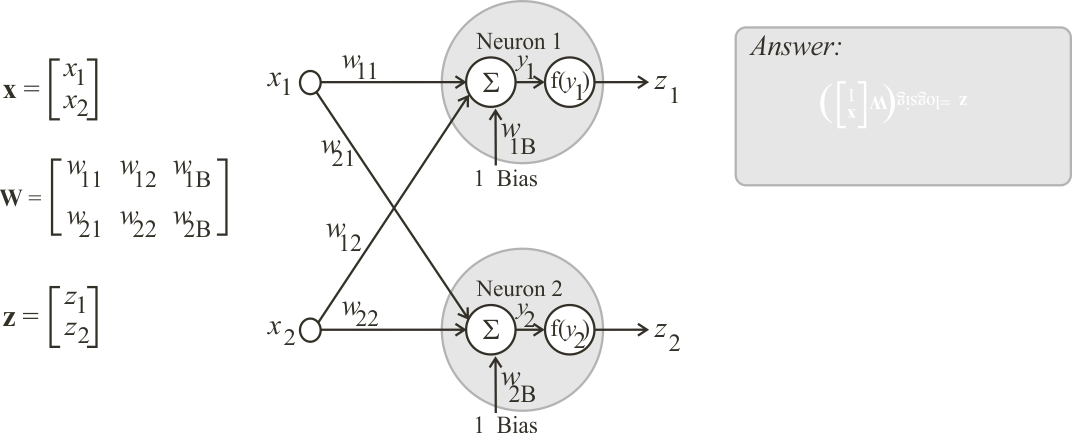Problem 14 Compute the output value for the neural network shown below (perform the computation manually). The ANN has two inputs, two neurons in the hidden layer 1, one neuron in the output layer and one output. Suppose that the ANN is using the logsig function.Problem 15 Repeat the last problem using Neural Lab code your project name must be TwoLayer. After running the program, double click on the z to see the value of the output of the network.Problem 16 Search over the Internet for other activation functions besides the logsig and tanh.

 Problem 17 An ANN was implemented by hardware as shown. A power failure damaged the weight w12. Before the power failure problem, the output network was 0.92129 when the input x was applied. Compute the value of the weight w12 for the network to operate normally. Suppose the activation function is the logsig.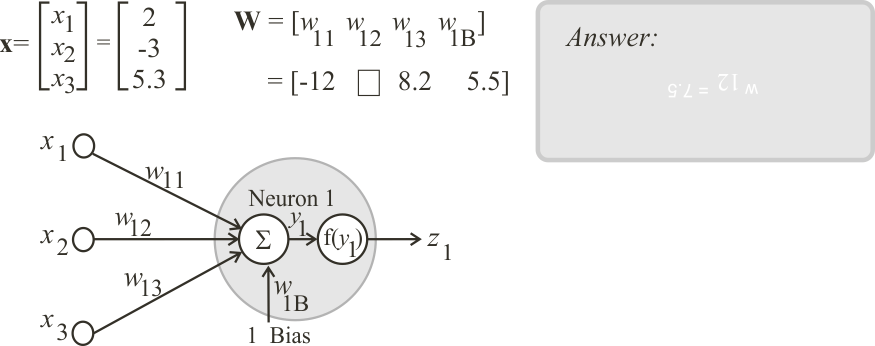Problem 18 An ANN was implemented by hardware as shown. A power failure damaged the weights w11 and w12. Before the problem, the output network was 0.539915 when the first column of x was applied, and 0.327393 for the second column. Compute the values of the weights w11 and w12 for the network to operate appropriately.Problem 19 The network of the figure has two hidden layers. (a) Compute the size of the matrices: W1, W2 and W3. (b) Compute the number of weights of this ANN.